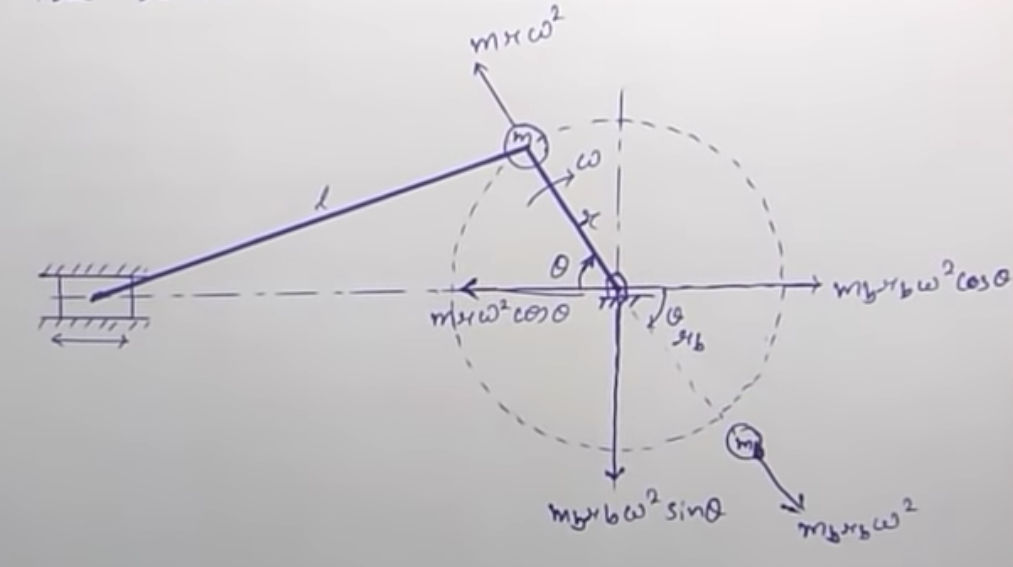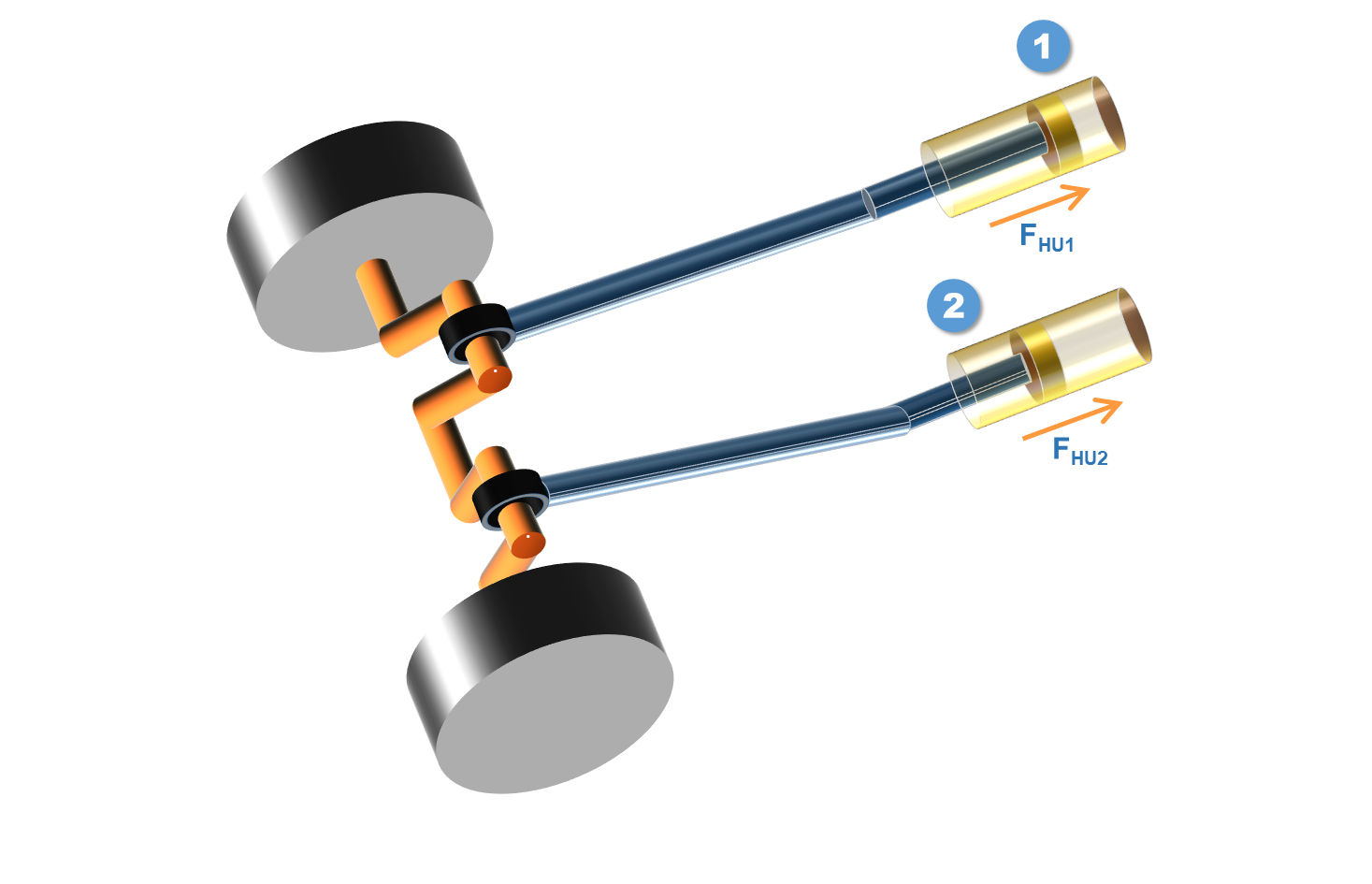Variation of Tractive Force | Tractive Effort | Effect of Partial Balancing of Locomotives

We have studied in earlier video that balancing is done on a locomotive. But because of balancing there are 3 effects that occurs on the locomotive, which are:

1. Variation of tractive force
2. Swaying couple
3. Hammer blow

In this notes we will study the effect of variation of tractive force and also derive the equation to calculate the tractive force.

The first thing to remember here is that the Locomotive engines are reciprocating engines and we know that we cannot completely balance a reciprocating engine, we partially balance it. And partially balancing generates horizontal force and vertical force.In the video of partial balancing of reciprocating of engines ( Video Part-1 & Video Part-2 ) we have studied that the unbalanced force acting in horizontal and vertical direction is given by:

\begin{aligned} F_H &= (1-C) \sdot mr \omega^2 \sdot \cos \theta \ \ --- (1) \\ F_V &= C \sdot mr \omega^2 \sdot \sin \theta \end{aligned}

Now, let us imagine the horizontal force $F_H$ in three dimensions by looking at this diagramUnbalanced horizontal force acting on cylinders of locomotive

In the above diagram, let's represent the horizontal unbalanced force acting on cylinder 1 as $F_{HU1}$ and similarly the horizontal unbalanced force acting on cylinder 2 as $F_{HU2}$.

From this, it becomes easier to understand what is traction force. It is the resultant of the two horizontal unbalanced force. Hence we get the following equation:

$F_T = F_{HU1} + F_{HU2} \ \ --- (2)$Traction force definition

The resultant unbalanced horizontal force due to the two cylinder along the line of stroke of cylinder is called traction force.

Remember:

Try to write the definition by explaining the things that we did in the calculation. This way it becomes easier for you to understand it.

Now for cylinder 1, and using equation (1) from above, we can say that:

$F_{HU1} = (1-C) \sdot mr\omega^2 \sdot \cos \theta \ \ --- (3)$

Similarly for cylinder 2:

$F_{HU2} = (1-C) \sdot mr\omega^2 \sdot \cos \theta \ \ --- (4)$

As we have studied in the last video, if cylinder 1 angle is at $\theta$ then the cylinder 2 angle will be at $\theta + 90\degree$. Therefore from equation (4) we can say that:

$F_{HU2} = (1-C) \sdot mr\omega^2 \sdot \cos (\theta + 90\degree) \ \ --- (5)$

From equation (2)

\begin{aligned} T_F &= F_{HU1} + F_{HU2} \\ &= (1-C) \sdot mr\omega^2 \sdot \cos \theta + (1-C) \sdot mr\omega^2 \sdot \cos (\theta + 90\degree) \\ &= (1-C) \sdot mr\omega^2 \sdot \big[\cos \theta + \cos (\theta + 90\degree) \big] \\ &= (1-C) \sdot mr\omega^2 \big[\cos \theta - \sin \theta \big] \ \ --- (6) \\ \\ where, \ &m = \text{mass of the reciprocating part} \\ &r = \text{radius of the crank} \\ &\omega = \text{angular velocity of the crank} \\ &C = \text{portion of the reciprocating part which is to be balanced} \\ \end{aligned}

Now we need to find the maximum and minimum of this tractive force. To do this we need to differentiate equation (6) with respect to the variables. Here our variable is $\theta$. So we need to differentiate $F_T$ with respect to $\theta$.

Doing this we get:

\begin{aligned} {dF_T \over d\theta} &= {d \over d\theta} \big[(1-C) \sdot mr\omega^2 \sdot (\cos \theta - \sin \theta) \big] = 0 \\ \therefore \ &(1-C) \sdot mr\omega^2 \sdot {d \over d\theta} (\cos \theta - \sin \theta) = 0 \\ \therefore \ &{d \over d\theta} (\cos \theta - \sin \theta) = 0 \\ \therefore \ &- \sin \theta - \cos \theta = 0 \\ \therefore \ &\sin \theta + \cos \theta = 0 \\ \therefore \ &\sin \theta = - \cos \theta \\ \therefore \ &\tan \theta = -1 \end{aligned}

Therefore, we get $\theta = 135\degree$ or $315\degree$.

Now we will put the value of both these $\theta$ in equation (6) to find the variation of tractive force.

For $\theta = 135\degree$ we get,

\begin{aligned} F_T &= (1-C) \sdot mr\omega^2 \sdot (\cos 135\degree - \sin 135\degree) \\ & \boxed {\therefore F_{T(min)} = -\sqrt2 \sdot (1-C) \sdot mr\omega^2} \end{aligned}

Similarly for $\theta = 315\degree$ we get,

\begin{aligned} F_T &= (1-C) \sdot mr\omega^2 \sdot (\cos 315\degree - \sin 315\degree) \\ & \boxed {\therefore F_{T(max)} = \sqrt2 \sdot (1-C) \sdot mr\omega^2} \end{aligned}

Since we get the maximum and minimum value of tractive force, we can say that the value of $T_F$ varies between $\sqrt2 \sdot (1-C) \sdot mr\omega^2$ to $-\sqrt2 \sdot (1-C) \sdot mr\omega^2$.

Which we can represent as:

$\boxed{F_T = \pm \sqrt2 \sdot (1-C) \sdot mr\omega^2}$